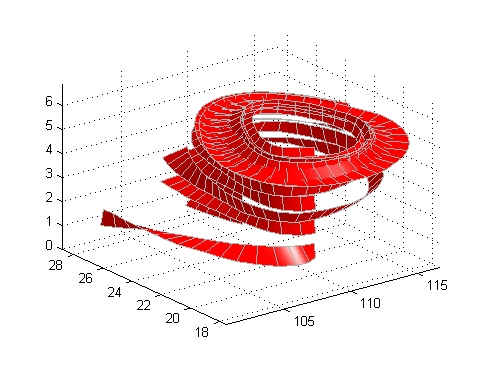3-D VisualizationDisplaying Curl with Stream Ribbons

Stream ribbons illustrate direction of flow, similar to stream lines, but can also show rotation about the flow axis by twisting the ribbon-shaped flow line. The `streamribbon` function enables you to specify a twist angle (in radians) for each vertex in the stream ribbons.

When used in conjunction with the `curl` function, `streamribbon` is useful for displaying the curl angular velocity of a vector field. The following example illustrates this technique:

1. Select a Subset of Data to Plot

Load and select a region of interest in the `wind` data set using `subvolume`. Plotting the full data set first can help you select a region of interest.

• ```load wind
lims = [100.64 116.67 17.25 28.75 -0.02 6.86];
[x,y,z,u,v,w] = subvolume(x,y,z,u,v,w,lims);
```

2. Calculate Curl Angular Velocity and Wind Speed

Calculate the `curl` angular velocity and the wind speed.

• ```cav = curl(x,y,z,u,v,w);
wind_speed = sqrt(u.^2 + v.^2 + w.^2);
```

3. Create the Stream Ribbons

• Use `meshgrid` to create arrays of starting points for the stream ribbons. See Starting Points for Stream Plots in this chapter for information on specifying the arrays of starting points.
• `stream3` calculates the stream line vertices with a step size of `.5`.
• `streamribbon` scales the width of the ribbon by a factor of `2` to enhance the visibility of the twisting (which indicates curl angular velocity).
• `streamribbon` returns the handles of the surface objects it creates, which are then used to set the color to red (`FaceColor`), the color of the surface edges to light gray (`EdgeColor`), and slightly increase the brightness of the ambient light reflected when lighting is applied (`AmbientStrength`).
• ```[sx sy sz] = meshgrid(110,20:5:30,1:5);
verts = stream3(x,y,z,u,v,w,sx,sy,sz,.5);
h = streamribbon(verts,x,y,z,cav,wind_speed,2);
set(h,'FaceColor','r',...
'EdgeColor',[.7 .7 .7],...
'AmbientStrength',.6)
```

4. Define the View and Add Lighting

• The `volumebounds` command provides a convenient way to set `axis` and color limits.
• Add a `grid` and set the `view` for 3-D (`streamribbon` does not change the current view).
• `camlight` creates a light positioned to the right of the viewpoint and `lighting` sets the lighting method to Phong (which requires the Z-buffer renderer).
• ```axis(volumebounds(x,y,z,wind_speed))
grid on
view(3)
camlight right;
set(gcf,'Renderer','zbuffer'); lighting phong```Stream Line Plots of Vector Data Displaying Divergence with Stream Tubes© 1994-2005 The MathWorks, Inc.